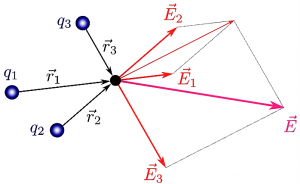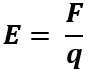Physics

# Electric field

The electric field is any spatial area where the electric charges, which can be positive or negative, are related in a certain space limit, being the electric field both the delimitation of the space in the plane and the space of a body charged with electricity. It is not measurable in itself, but what is measured is the effect generated by electric charges inside. In 1832, Faraday proposed the idea of the electric field in greater depth to demonstrate the principle of electromagnetic induction.Related Topics

Electric charge, electrostatic field, magnetic field

## What is an electric field?

The electric field is defined as the space sector designated from the electric force, which is made up of two or more charges. The direction taken by the electric field is influenced by the force direction it will exert on a positive charge. It is generated radially towards the outside of a positive charge and radially towards the inside of being a point charge.

## Parts

• Magnitude (Intensity): defined as the vector size that represents the field. A model of particles found within the electric field is used.
• Direction: visualized by an axis that crosses the particles and the point in space that is in interaction.
• Sense: it is determined from the line orientation that represents the magnitude located on the axis that provides the direction of the electric field.
• Electric field lines or force lines: these are defined from imaginary lines that are drawn in such a way that their direction at any point is the same as the direction of the field at that point. They move away in positive electrical charges and move away in negative electrical charges.
• Force: The electric force is the set that exists between the electric charges.

## Characteristics of electric field

Electric fields must have the following characteristics for proper operation:

• They depend solely on the load that generates it.
• The electric field exists only with the detection of one electric charge, the second or other charges are not necessary for its detection. Just as it exists even if the load is not moving.
• Two or more electrical charges interact, which can be positive (+) or negative (-). Opposite charges are attracted, and equal charges are repelled.
• The visualization of the lines in the electric field manages to visualize the magnitude and direction of E.
• The intensity or magnitude of the electric field is measured in volts per meter (v/m). This decreases as the distance from the source increases.
• The energy source is based on the electrical voltage.

## History

For the understanding of electricity operation during the 18th century, researches such as Coulomb’s, focus on electricity and its internal and external functioning and its handling in everyday use. It was established that electric charges could interact at the distance between them, as well as masses against gravitational forces. That is to say, that a mass or a charge could inexplicably, without any mediator, notice the presence of another in its surroundings.

Faraday proposes an alternative interpretation, which will be very useful, contributing to the idea of the electric field, the proposal that: the space surrounding an electric charge is affected by its presence, since it modifies its characteristics. In one of his attempts to test his approach, Faraday describes it as invisible tentacles advancing from an electric charge.

In this way, from the moment a body acquires a charge, this information spreads rapidly in its environment, in fact, at the speed of light, and can eventually reach another charge. Subsequently, the interaction between one charge and another is discussed, as it is possible to describe and study the fact from the interaction of charges in the field where it interacts.

In order to represent the electric field, the force lines are used, which would be the vectors that leave the positive electric charges to enter the negative charges. In this way, the direction of the force of the electric field is indicated, which in turn would experiment a punctual and positive charge, called the test charge. The number of force lines would be proportional to the intensity of the electric field, since when counting on the interaction lines they are very close, the field is large and where they are separated, the field is small.

Researchers such as Michael Faraday, and later studies by James Clerk Maxwell, allowed the first descriptions of electrical phenomena, for example, Coulomb’s law, where only electrical charges were taken into account; to then develop more complete laws where field variation is studied.

## What is an electric field for?

The electric field serves to highlight the interaction of charged particles, and to determine the force on a charge located at that point.

## Electric field units

The unit of measurement for electric field strength or magnitude is used in volts per meter (v/m).

The Coulomb law can be used in the case of electric fields, because it establishes how the force is between two punctual electric charges, being a punctual electric charge, the charge that is located at a geometric point at a spatial level. Remembering that it would be used in specific cases, given that these charges must be at rest, generating electrostatics. This law studies the interaction between electrical charges that are particularly small compared to the distance between them.

## Formula

The formula for calculating the electric field is as follows:Where E is Electric field strength, F is Strength and q is Load.

## Types

There are three types of electric fields. The first is produced by a charge distribution and is known as an electrostatic field. The second and third are associated with two types of magnetic induction, one produced by spatial movement with respect to the magnetic flux and the other produced by the bonding time that modifies the magnetic flux.

## Uniform electric field

The electric field consists of the space zone where the electric charges interact, being these of the same magnitude, sense and direction. For example, by having two charged parallel plates, the electric field will be oriented from the plate with a positive electric charge to the plate with a negative charge, generating that the vector does not change.

## Examples

• Electric Lamps: An electric lamp when plugged into a current by an electric cable is able to generate electric fields in the air surrounding that device. The higher the voltage, the greater the intensity of the electric field. Voltage can exist even if there is no active electric current, since it is not required that the electrical appliance is active for an electric field to be generated around it.
• Radio and television transmitting and receiving antennas: From a transmitting device of a radio station towards a common radio, the message is transferred through an electric field, which is generated from antennas that capture the information and transmit it. Its most common shape would be a metal rod. Each radio station has certain frequency, which generate different electric fields, the operation is given from the periodic movement of electric energy charges, which move from one end to another, generating at one end of the antenna an excess of negative charge while at the other end, generates a positive charge deficit, are exchanged from one end to the other generating polarity.
Written by Gabriela Briceño V.(3 votos, promedio: 5.00 de 5)Loading...